Next: Application: Symmetric Enclosure versus Up: Selective Symmetry Detection for Previous: Projecting Edges onto the

## Symmetric Enclosure

After noting the edges intersecting the template and attenuating them appropriately, the edges are sorted into 32 angular bins depending on the region of the template they fall under. Figuredisplays the division of the model by a ray emanating from its center at 32 angular intervals to form these bins. Within each bin i, the strongest projected edge magnitude is stored as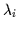. In this way, we form an angular profile of the edge data as shown in Figure. Symmetric enclosure is then computed by summing the contribution to symmetry of each pair of bins as in Equationwhich is derived from Equation: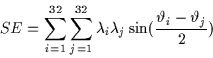(2.9)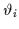Angular position of ith binPeak projected edge magnitude in ith bin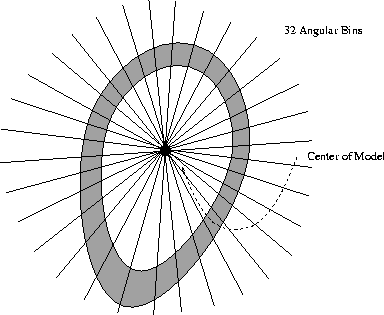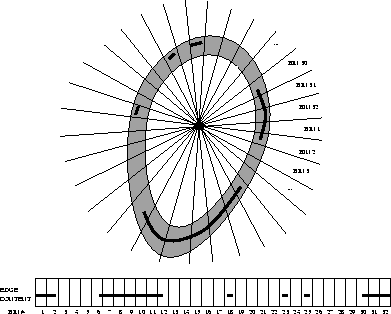Due to the pixelization of the edge data, smaller templates will not overlap enough pixels in the edge map to trigger each of their 32 angular bins. Thus, small templates will yield consistently lower values of SE. Therefore each template's output is normalized and presented as a percentage of the possible peak output of the template. For each template, we compute the maximum possible value of SE. The final output is a value from 0 to 100% allowing the implementation of a template-independent threshold on SE. Thus, resolution variations in the image due to scaling and discretization will not affect the response of our template-based symmetry detection.Next: Application: Symmetric Enclosure versus Up: Selective Symmetry Detection for Previous: Projecting Edges onto the
Tony Jebara
2000-06-23